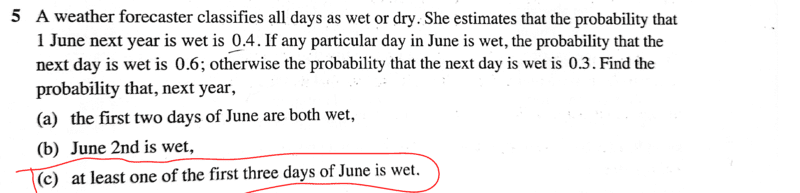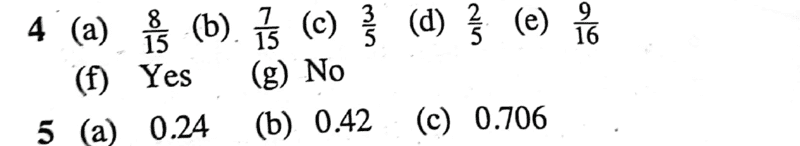# Solve the given problem that involves Probability

Gold Member
Homework Statement:
See attached;
Relevant Equations:
Probability
I may seek an alternative approach; actually i had thought that this would take a few minutes of my time..but just realized that it just takes a minute; My interest is only on highlighted part.Text solutionMy take;

##P(\text{at least one of the first three days is wet})=1-P(ddd)##
=## 1-(0.6×0.7×0.7)=0.706##

Of course the other way of doing it would also realize the same result but will need more time...i.e using
##P(www)+P(wwd)+P(wdw)+P(wdd)+P(dww)+...P(ddw)=0.706##

Cheers man!

Last edited by a moderator:

Mentor
My take;
##P(\text{at least one of the first three days is wet})=1-P(ddd)##
=## 1-(0.6×0.7×0.7)=0.706##
IMO, the approach above is the better approach. It uses the idea that the complement of "at least one day of the three is wet" is "none of the three days is wet." The probabilities of these two events has to add to 1.
chwala said:
Of course the other way of doing it would also realize the same result but will need more time...i.e using
##P(www)+P(wwd)+P(wdw)+P(wdd)+P(dww)+...P(ddw)=0.706##
The latter approach is trickier to get right in that you have to ensure that you have included all possible events in which one, two, or three of the days is wet. In the probabilities that you list, you have omitted one. These come from the following combinations.
3 wet days: ##\binom {3}{3} = 1## -- www
2 wet days: ##\binom{3}{2} = 3## -- wwd, wdw, dww
1 wet day: ##\binom{3}{1} - 3## -- wdd, dwd, ddw

•chwala
Gold Member
IMO, the approach above is the better approach. It uses the idea that the complement of "at least one day of the three is wet" is "none of the three days is wet." The probabilities of these two events has to add to 1.
The latter approach is trickier to get right in that you have to ensure that you have included all possible events in which one, two, or three of the days is wet. In the probabilities that you list, you have omitted one. These come from the following combinations.
3 wet days: ##\binom {3}{3} = 1## -- www
2 wet days: ##\binom{3}{2} = 3## -- wwd, wdw, dww
1 wet day: ##\binom{3}{1} - 3## -- wdd, dwd, ddw
Actually, I didn't omit...I just indicated the sum total from the first to last term... would yield same result. Apologies for confusion.